## 5.358. soft_all_equal_min_var

Origin
Constraint

$\mathrm{𝚜𝚘𝚏𝚝}_\mathrm{𝚊𝚕𝚕}_\mathrm{𝚎𝚚𝚞𝚊𝚕}_\mathrm{𝚖𝚒𝚗}_\mathrm{𝚟𝚊𝚛}\left(𝙽,\mathrm{𝚅𝙰𝚁𝙸𝙰𝙱𝙻𝙴𝚂}\right)$

Arguments
 $𝙽$ $\mathrm{𝚍𝚟𝚊𝚛}$ $\mathrm{𝚅𝙰𝚁𝙸𝙰𝙱𝙻𝙴𝚂}$ $\mathrm{𝚌𝚘𝚕𝚕𝚎𝚌𝚝𝚒𝚘𝚗}\left(\mathrm{𝚟𝚊𝚛}-\mathrm{𝚍𝚟𝚊𝚛}\right)$
Restrictions
 $𝙽\ge 0$ $\mathrm{𝚛𝚎𝚚𝚞𝚒𝚛𝚎𝚍}$$\left(\mathrm{𝚅𝙰𝚁𝙸𝙰𝙱𝙻𝙴𝚂},\mathrm{𝚟𝚊𝚛}\right)$
Purpose

Let $M$ be the number of occurrences of the most often assigned value to the variables of the $\mathrm{𝚅𝙰𝚁𝙸𝙰𝙱𝙻𝙴𝚂}$ collection. $𝙽$ is greater than or equal to the total number of variables of the $\mathrm{𝚅𝙰𝚁𝙸𝙰𝙱𝙻𝙴𝚂}$ collection minus $M$ (i.e., $𝙽$ is greater than or equal to the minimum number of variables that need to be reassigned in order to obtain a solution where all variables are assigned a same value).

Example
$\left(1,〈5,1,5,5〉\right)$

Within the collection $〈5,1,5,5〉$, 3 is the number of occurrences of the most assigned value. Consequently, the $\mathrm{𝚜𝚘𝚏𝚝}_\mathrm{𝚊𝚕𝚕}_\mathrm{𝚎𝚚𝚞𝚊𝚕}_\mathrm{𝚖𝚒𝚗}_\mathrm{𝚟𝚊𝚛}$ constraint holds since the argument $𝙽=1$ is greater than or equal to the total number of variables 4 minus 3.

Typical
 $𝙽>0$ $𝙽<|\mathrm{𝚅𝙰𝚁𝙸𝙰𝙱𝙻𝙴𝚂}|$ $𝙽<|\mathrm{𝚅𝙰𝚁𝙸𝙰𝙱𝙻𝙴𝚂}|/10+2$ $|\mathrm{𝚅𝙰𝚁𝙸𝙰𝙱𝙻𝙴𝚂}|>1$
Symmetries
• $𝙽$ can be increased.

• Items of $\mathrm{𝚅𝙰𝚁𝙸𝙰𝙱𝙻𝙴𝚂}$ are permutable.

• All occurrences of two distinct values of $\mathrm{𝚅𝙰𝚁𝙸𝙰𝙱𝙻𝙴𝚂}.\mathrm{𝚟𝚊𝚛}$ can be swapped; all occurrences of a value of $\mathrm{𝚅𝙰𝚁𝙸𝙰𝙱𝙻𝙴𝚂}.\mathrm{𝚟𝚊𝚛}$ can be renamed to any unused value.

Algorithm

Let $m$ denote the total number of potential values that can be assigned to the variables of the $\mathrm{𝚅𝙰𝚁𝙸𝙰𝙱𝙻𝙴𝚂}$ collection. In [HebrardMarxSullivanRazgon09], E. Hebrard et al. provides an $O\left(m\right)$ filtering algorithm achieving arc-consistency on the $\mathrm{𝚜𝚘𝚏𝚝}_\mathrm{𝚊𝚕𝚕}_\mathrm{𝚎𝚚𝚞𝚊𝚕}_\mathrm{𝚖𝚒𝚗}_\mathrm{𝚟𝚊𝚛}$ constraint. The same paper also provides an algorithm with a lower complexity for achieving range consistency. Both algorithms are based on the following ideas:

• In a first phase, they both compute an envelope of the union $𝒟$ of the domains of the variables of the $\mathrm{𝚅𝙰𝚁𝙸𝙰𝙱𝙻𝙴𝚂}$ collection, i.e., an array $A$ that indicates for each potential value $v$ of $𝒟$, the maximum number of variables that could possibly be assigned value $v$. Let $\mathrm{𝑚𝑎𝑥}_\mathrm{𝑜𝑐𝑐}$ denote the maximum value over the entries of array $A$, and let ${𝒱}_{\mathrm{𝑚𝑎𝑥}}_\mathrm{𝑜𝑐𝑐}$ denote the set of values which all occur in $\mathrm{𝑚𝑎𝑥}_\mathrm{𝑜𝑐𝑐}$ variables of the $\mathrm{𝚅𝙰𝚁𝙸𝙰𝙱𝙻𝙴𝚂}$ collection. The quantity $|\mathrm{𝚅𝙰𝚁𝙸𝙰𝙱𝙻𝙴𝚂}|-\mathrm{𝑚𝑎𝑥}_\mathrm{𝑜𝑐𝑐}$ is a lower bound of $𝙽$.

• In a second phase, depending on the relative ordering between $\mathrm{𝑚𝑎𝑥}_\mathrm{𝑜𝑐𝑐}$ and the minimum value of $|\mathrm{𝚅𝙰𝚁𝙸𝙰𝙱𝙻𝙴𝚂}|-𝙽$, i.e., $|\mathrm{𝚅𝙰𝚁𝙸𝙰𝙱𝙻𝙴𝚂}|-\overline{𝙽}$, we have the three following cases:

1. When $\mathrm{𝑚𝑎𝑥}_\mathrm{𝑜𝑐𝑐}<|\mathrm{𝚅𝙰𝚁𝙸𝙰𝙱𝙻𝙴𝚂}|-\overline{𝙽}$, the constraint $\mathrm{𝚜𝚘𝚏𝚝}_\mathrm{𝚊𝚕𝚕}_\mathrm{𝚎𝚚𝚞𝚊𝚕}_\mathrm{𝚖𝚒𝚗}_\mathrm{𝚟𝚊𝚛}$ simply fails since not enough variables of the $\mathrm{𝚅𝙰𝚁𝙸𝙰𝙱𝙻𝙴𝚂}$ collection can be assigned the same value.

2. When $\mathrm{𝑚𝑎𝑥}_\mathrm{𝑜𝑐𝑐}=|\mathrm{𝚅𝙰𝚁𝙸𝙰𝙱𝙻𝙴𝚂}|-\overline{𝙽}$, the constraint $\mathrm{𝚜𝚘𝚏𝚝}_\mathrm{𝚊𝚕𝚕}_\mathrm{𝚎𝚚𝚞𝚊𝚕}_\mathrm{𝚖𝚒𝚗}_\mathrm{𝚟𝚊𝚛}$ can be satisfied. In this context, a value $v$ can be removed from the domain of a variable $V$ of the $\mathrm{𝚅𝙰𝚁𝙸𝙰𝙱𝙻𝙴𝚂}$ collection if and only if:

1. value $v$ does not belong to ${𝒱}_{\mathrm{𝑚𝑎𝑥}}_\mathrm{𝑜𝑐𝑐}$,

2. the domain of variable $V$ contains all values of ${𝒱}_{\mathrm{𝑚𝑎𝑥}}_\mathrm{𝑜𝑐𝑐}$.

On the one hand, the first condition can be understand as the fact that value $v$ is not a value that allows to have at least $|\mathrm{𝚅𝙰𝚁𝙸𝙰𝙱𝙻𝙴𝚂}|-\overline{𝙽}$ variables assigned the same value. On the other hand, the second condition can be interpreted as the fact that variable $V$ is absolutely required in order to have at least $|\mathrm{𝚅𝙰𝚁𝙸𝙰𝙱𝙻𝙴𝚂}|-\overline{𝙽}$ variables assigned the same value.

3. When $\mathrm{𝑚𝑎𝑥}_\mathrm{𝑜𝑐𝑐}>|\mathrm{𝚅𝙰𝚁𝙸𝙰𝙱𝙻𝙴𝚂}|-\overline{𝙽}$, the constraint $\mathrm{𝚜𝚘𝚏𝚝}_\mathrm{𝚊𝚕𝚕}_\mathrm{𝚎𝚚𝚞𝚊𝚕}_\mathrm{𝚖𝚒𝚗}_\mathrm{𝚟𝚊𝚛}$ can be satisfied, but no value can be pruned.

Note that, in the context of range consistency, the first phase of the filtering algorithm can be interpreted as a sweep algorithm were:

• On the one hand, the sweep status corresponds to the maximum number of occurrence of variables that can be assigned a given value.

• On the other hand, the event point series correspond to the minimum values of the variables of the $\mathrm{𝚅𝙰𝚁𝙸𝙰𝙱𝙻𝙴𝚂}$ collection as well as to the maximum values ($+1$) of the same variables.

##### Figure 5.358.1. Illustration of the two phases filtering algorithm of the $\mathrm{𝚜𝚘𝚏𝚝}_\mathrm{𝚊𝚕𝚕}_\mathrm{𝚎𝚚𝚞𝚊𝚕}_\mathrm{𝚖𝚒𝚗}_\mathrm{𝚟𝚊𝚛}$$\left(1,〈{V}_{1},{V}_{2},{V}_{3},{V}_{4}〉\right)$ constraint with ${V}_{1}\in \left[1,3\right]$, ${V}_{2}\in \left[3,7\right]$, ${V}_{3}\in \left[0,8\right]$ and ${V}_{4}\in \left[5,6\right]$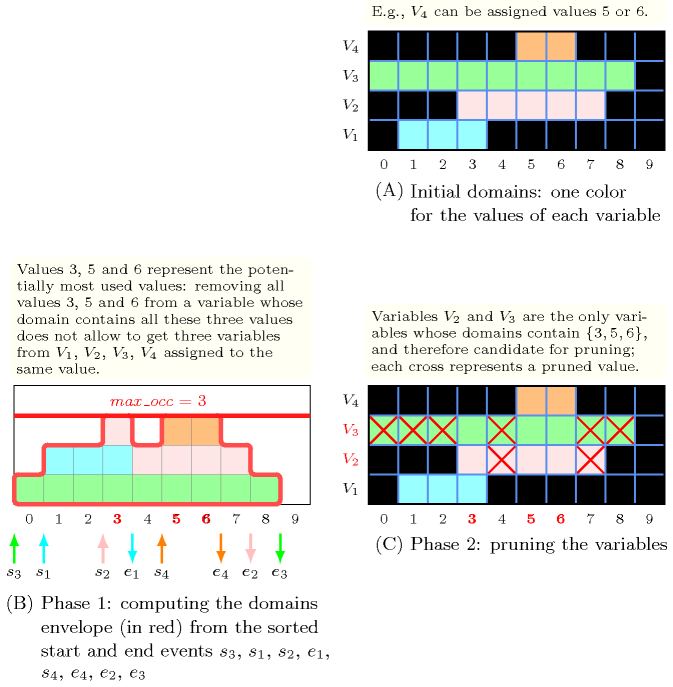Figure 5.358.1 illustrates the previous filtering algorithm on an example where $𝙽$ is equal to 1, and where we have four variables ${V}_{1}$, ${V}_{2}$, ${V}_{3}$ and ${V}_{4}$ respectively taking their values within intervals $\left[1,3\right]$, $\left[3,7\right]$, $\left[0,8\right]$ and $\left[5,6\right]$ (see Part (A) of Figure 5.358.1, where the values of each variable are assigned a same colour that we retrieve in the other parts of Figure 5.358.1).

Part (B) of Figure 5.358.1 illustrates the first phase of the filtering algorithm, namely the computation of the envelope of the domains of variables ${V}_{1}$, ${V}_{2}$, ${V}_{3}$ and ${V}_{4}$. The start events ${s}_{1}$, ${s}_{2}$, ${s}_{3}$, ${s}_{4}$ (i.e., the events respectively associated with the minimum value of variables ${V}_{1}$, ${V}_{2}$, ${V}_{3}$, ${V}_{4}$) where the envelope is increased by 1 are represented by the character $↑$. Similarly, the end events (i.e., the events ${e}_{1}$, ${e}_{2}$, ${e}_{3}$, ${e}_{4}$ respectively associated with the maximum value ($+1$) of ${V}_{1}$, ${V}_{2}$, ${V}_{3}$, ${V}_{4}$ are represented by the character $↓$). Since the highest peak of the envelope is equal to 3 we have that $\mathrm{𝑚𝑎𝑥}_\mathrm{𝑜𝑐𝑐}$ is equal to 3. The values that allow to reach this highest peak are equal to ${𝒱}_{\mathrm{𝑚𝑎𝑥}}_\mathrm{𝑜𝑐𝑐}=\left\{3,5,6\right\}$ (i.e., shown in red in Part (B) of Figure 5.358.1).

Finally, Part (C) of Figure 5.358.1 illustrates the second phase of the filtering algorithm. Since $\mathrm{𝑚𝑎𝑥}_\mathrm{𝑜𝑐𝑐}=3$ is equal to $|\mathrm{𝚅𝙰𝚁𝙸𝙰𝙱𝙻𝙴𝚂}|-\overline{𝙽}=4-1$ we remove from the variables whose domains contain ${𝒱}_{\mathrm{𝑚𝑎𝑥}}_\mathrm{𝑜𝑐𝑐}=\left\{3,5,6\right\}$ (i.e., variables ${V}_{2}$ and ${V}_{3}$) all values not in ${𝒱}_{\mathrm{𝑚𝑎𝑥}}_\mathrm{𝑜𝑐𝑐}=\left\{3,5,6\right\}$ (i.e., values 4, 7 for variable ${V}_{2}$ and values 0, 1, 2, 4, 7, 8 for variable ${V}_{3}$).

Counting
 Length ($n$) 2 3 4 5 6 7 8 Solutions 21 172 1845 24426 386071 7116320 150156873

Number of solutions for $\mathrm{𝚜𝚘𝚏𝚝}_\mathrm{𝚊𝚕𝚕}_\mathrm{𝚎𝚚𝚞𝚊𝚕}_\mathrm{𝚖𝚒𝚗}_\mathrm{𝚟𝚊𝚛}$: domains $0..n$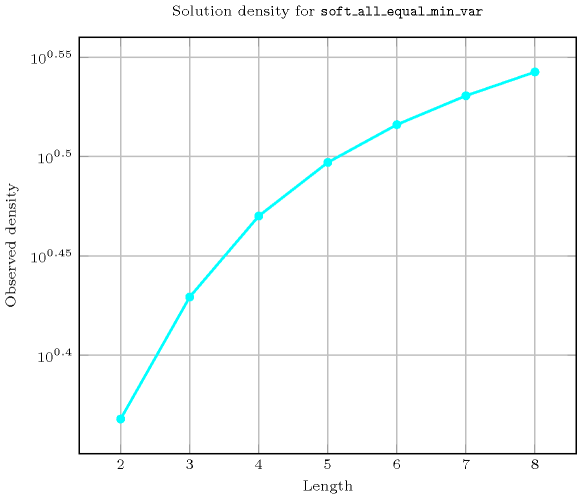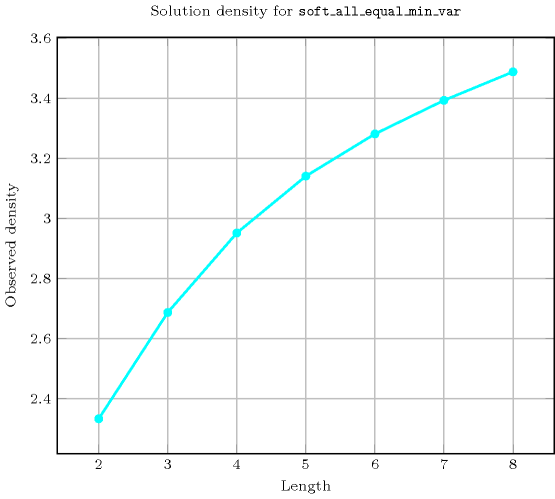Length ($n$)2345678
Total211721845244263860717116320150156873
 Parameter value

03456789
194085156259400585
296450516564039863216713
3-64625705633859104672274761
4--62577761126097514722852721
5---7776117649205683218234801
6----117649209715242683841
7-----209715243046721
8------43046721

Solution count for $\mathrm{𝚜𝚘𝚏𝚝}_\mathrm{𝚊𝚕𝚕}_\mathrm{𝚎𝚚𝚞𝚊𝚕}_\mathrm{𝚖𝚒𝚗}_\mathrm{𝚟𝚊𝚛}$: domains $0..n$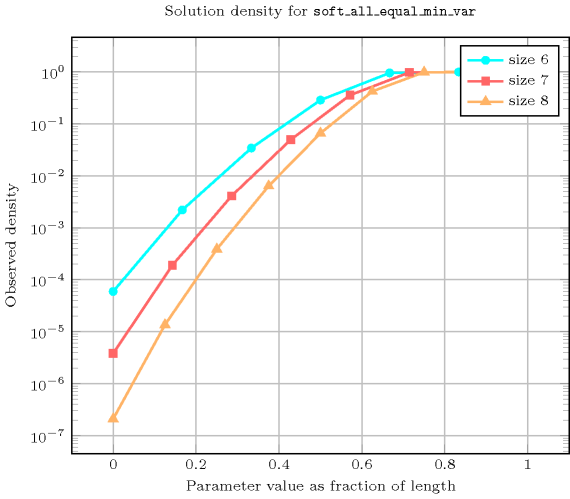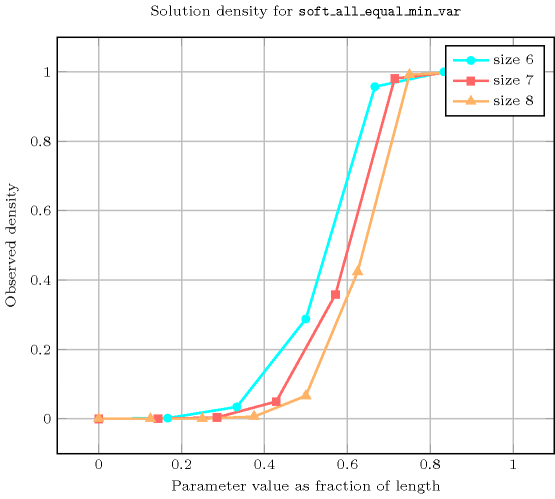Keywords
Arc input(s)

$\mathrm{𝚅𝙰𝚁𝙸𝙰𝙱𝙻𝙴𝚂}$

Arc generator
$\mathrm{𝐶𝐿𝐼𝑄𝑈𝐸}$$↦\mathrm{𝚌𝚘𝚕𝚕𝚎𝚌𝚝𝚒𝚘𝚗}\left(\mathrm{𝚟𝚊𝚛𝚒𝚊𝚋𝚕𝚎𝚜}\mathtt{1},\mathrm{𝚟𝚊𝚛𝚒𝚊𝚋𝚕𝚎𝚜}\mathtt{2}\right)$

Arc arity
Arc constraint(s)
$\mathrm{𝚟𝚊𝚛𝚒𝚊𝚋𝚕𝚎𝚜}\mathtt{1}.\mathrm{𝚟𝚊𝚛}=\mathrm{𝚟𝚊𝚛𝚒𝚊𝚋𝚕𝚎𝚜}\mathtt{2}.\mathrm{𝚟𝚊𝚛}$
Graph property(ies)
$\mathrm{𝐌𝐀𝐗}_\mathrm{𝐍𝐒𝐂𝐂}$$\ge |\mathrm{𝚅𝙰𝚁𝙸𝙰𝙱𝙻𝙴𝚂}|-𝙽$

Graph model

We generate an initial graph with binary equalities constraints between each vertex and its successors. The graph property states that $𝙽$ is greater than or equal to the difference between the total number of vertices of the initial graph and the number of vertices of the largest strongly connected component of the final graph.

Parts (A) and (B) of Figure 5.358.2 respectively show the initial and final graph associated with the Example slot. Since we use the $\mathrm{𝐌𝐀𝐗}_\mathrm{𝐍𝐒𝐂𝐂}$ graph property we show one of the largest strongly connected components of the final graph.

##### Figure 5.358.2. Initial and final graph of the $\mathrm{𝚜𝚘𝚏𝚝}_\mathrm{𝚊𝚕𝚕}_\mathrm{𝚎𝚚𝚞𝚊𝚕}_\mathrm{𝚖𝚒𝚗}_\mathrm{𝚟𝚊𝚛}$ constraint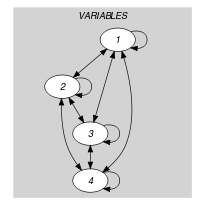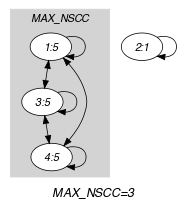(a) (b)